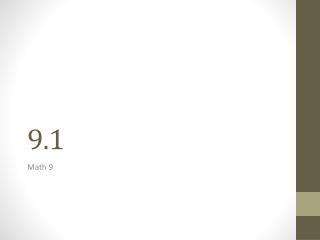DownloadDownload Presentation9 .1

# 9 .1

Download Presentation## 9 .1

- - - - - - - - - - - - - - - - - - - - - - - - - - - E N D - - - - - - - - - - - - - - - - - - - - - - - - - - -
##### Presentation Transcript

1. 9.1 Math 9

2. Do the “Math Link” with a partner on page 339. Discuss in class. 1.a) Each gondola could hold a number of people that is less than or equal to 60 1.b) The first Ferris wheel could hold a number of people that is less than or equal to 2160 3.a) Amount of weight it can hold. Size of seats can only hold so many people. 3.b) Height of person. Age of person. Medical condition.

3. Linear inequalities are a way to compare quantities to analyze situations. An inequality is: a mathematical statement comparing expressions that may not be equal.

4. Inequalities can be expressed three ways:

5. Use aclosed circle to represent this inequality Inequalities Expressed as Number Lines: Represent x ≤ −5 on a number line Represent x > −5 on a number line Use an open circle to represent this inequality

6. The boundary point separates the values less than from the values greater than the specified value An open circle shows that the boundary point is not includedin the solution. A closed circle shows that the boundary point is included in the solution.

7. Example 1 - In many provinces, you must be at least 16 years old to get a driver’s license. a) Sketch a number line to represent this situation. b) Represent this situation algebraically. Using a to represent age in years, a ≥ 16.

8. Example 2 – Expressing Inequalities a) Express the inequality shown algebraically X > 4

9. Example 2 – Expressing Inequalities b) Represent n < -12 on a number line

10. Example 2 – Expressing Inequalities c) Write an inequality for the values shownon the number line 2 ≥ X > -6 or 2 ≥ X and X > -6 or x ≤ 2 and x > -6

11. Example 2 – Expressing Inequalities d) Show the possible values for x on a number line, if -7 ≥ x . *What is a different way to express −7 ≥ x algebraically? X ≤ -7 2x ≤ -14

12. Example 3 – Represent a Combination of Inequalities Represent the temperature change from -19°C to 22°C both graphically and algebraically Using t to represent temperature then the change in temperature is written as t ≥ -19 and t ≤ 22. Or -19 ≤ t ≤ 22

13. By the way we are half way through the course. Summer School Summer Fun

14. Tutors Student Math tutors Eric Marshall   604 807 4705    gr 8-10 math volunteer LexiNiiranen   604 857 0140    En Ma gr 8-11 \$10/hr Chris Lee               604 928 8548    all grades Gavin Weise     604 5328392     gr 8- 12         Dominic  Nickel 604 625 2126    gr 8- 11 James Lee               778 867 2457    gr 8 - 11        Wendy Xu                604 761 4068    gr 8 -11 Teacher tutors Mr Kennedy 604 534 0306         Mr. Coletsis 604 532 8955

15. April 19, 2012 9.1 Assignment Corey “The Starter” Schneider P346 #1, 5, 6, 8, 9, 11, 13, 15, 16, 17, 18, 21, 22 *23, *24, *25